# What is dx?

Calculus teacher: It reminds you that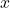is the variable in the integration.

Private math. tutor: Points will be deducted if one forgets to write it down.

Engineer: It is an infinitesimally small.

Teacher of advanced calculus: It is the differential of the function.

Measure theorist: We usually writeinstead.

Differential geometer: It is a smooth function mapping a point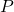in a manifold to an element in the cotangent space at.

Algebraic geometer: Let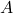be a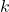-algebra over a field.is the equivalent classin the quotient, where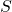is the-submodule ofgenerated by elements of the form. In general,is.

This entry was posted in math. Bookmark the permalink.